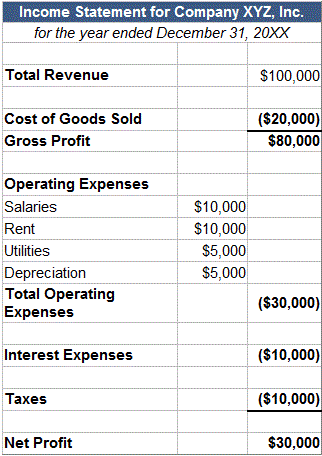## What is After-Tax Profit Margin?

After-tax profit margin is the percentage of revenue remaining after all operating expenses, interest, taxes and preferred stock dividends (but not common stock dividends) have been deducted from a company's total revenue.

## How Does After-Tax Profit Margin Work?

The formula for after-tax profit margin is:

(Total Revenue – Total Expenses)/Total Revenue = Net Profit/Total Revenue = After-Tax Profit Margin

By dividing net profit by total revenue, we can see what percentage of revenue made it all the way to the bottom line, which is good for investors.

Let's look at a hypothetical income statement for Company XYZ:Using the formula and the information above, we can calculate that Company XYZ's after-tax profit margin was \$30,000/\$100,000 = 30%

## Why Does After-Tax Profit Margin Matter?

After-tax profit margin is one of the most closely followed numbers in finance. Shareholders look at after-tax profit margin closely because it shows how good a company is at converting revenue into profits available for shareholders.

One of the most important concepts to understand is that net profit is not a measure of how much cash a company earned during a given period. This is because the income statement includes a lot of non-cash expenses such as depreciation and amortization. To learn about how much cash a company generates, you need to examine the cash flow statement.

Changes in after-tax profit margin are endlessly scrutinized. In general, when a company's after-tax profit margin is declining over time, a myriad of problems could be to blame, ranging from decreasing sales to poor customer experience to inadequate expense management.

After-tax profit margin is often used to compare companies within the same industry, in a process known as 'margin analysis.' After-tax profit margin is a percentage of sales, not an absolute number, so it can be extremely useful to compare after-tax profit margins among a group of companies to see which are most effective at converting sales into profits.

If you'd like to read more in-depth information about margin analysis, check out the following:

How to Use Margin Analysis as an Investment Tool -- learn how to use the three most common profit margin ratios to find the best investments.

Gross Profit Margin definition -- learn how gross profit margin is related to after-tax profit margin.

Operating Margin definition -- learn how gross profit margin is related to after-tax profit margin.